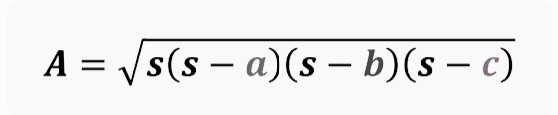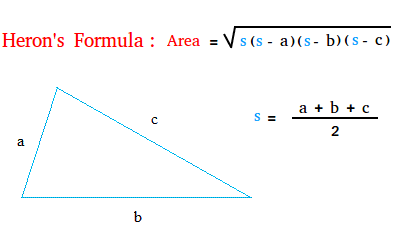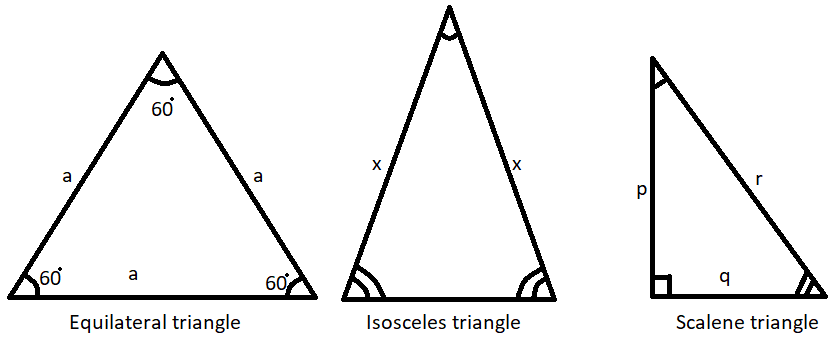Home   »   Scalene Triangle Definition, Formulas and Properties   »   Scalene Triangle Definition, Formulas and Properties

# Scalene Triangle- Definition, Properties, Area, Meaning

## Scalene Triangle

A scalene triangle is a type of geometric triangle that has three sides of different lengths and three angles of different measures. In other words, in a scalene triangle, all sides and angles are unequal. This is in contrast to an equilateral triangle, where all sides are of equal length, or an isosceles triangle, where at least two sides are of equal length.

In a scalene triangle:

1. No two sides are the same length.
2. No two angles are the same measure.
3. The sum of the interior angles is always 180 degrees.

## Scalene Triangle Meaning

A scalene triangle is one of the types of the triangle in which all three sides are different lengths. A scalene triangle’s angles have different measurements as well. We classified the types of triangles on the basis of side and the measurement of interior angles. Based on the sides, we can divide triangles into 3 classes- Scalene triangle, Equlileteral triangle, and Isosceles Triangle. In this article, we will learn about the Scalene Triangle Definition, formula, Angles, and many more. Let’s start.

## Scalene Triangle Definition

Before the Scalene triangle,we must know the definition of the Triangle.A triangle is a closed,two-dimensional geometric shape with three sides and angles in geometry.A scalene triangle is a triangle with three different side lengths and three different angle measurements.However, the sum of all the internal angles of the scalene triangle is unaffected by the various measurements. The sum of the scalene triangle’s three angles is always 180⁰

We can distinguish a scalene triangle from another triangle very easily because all three sides of scalene triangles are unequal and all the interior angles are differently measured.

## Properties of Scalene Triangle

Three sides of a scalene triangle have various lengths and angle measurements. Now, Give a look at the key properties of a scalene triangle.

1. Each of its three sides is of a different length.
2. There are three angles, and they are all of different sizes.
3. Its three inner angles add up to a total of 180 degrees.
4. It lacks rotational symmetry and points symmetry.
5. There is no rotational or point symmetry in it.
6. The angles within this triangle may be acute, obtuse, or right angles.
7. There is no line of symmetry because there are no parallel or equal sides

## Scalene Triangle Angles

The Scalene triangle’s interior angles might be right, acute, or obtuse angles.

• If all of the triangle’s interior angles are acute angle or less than 90 degrees (acute), then  The center of the circumscribing circle will be located inside a triangle
• If one of the interior angles of scalene triangles is obtuse or more than 60 then the circumcenter will be outside the scalene triangle.
• One of the triangle’s angles in a scalene triangle with right angles is equal to 90 degrees. therefore the midpoint of the hypotenuse is where the triangle’s circumcenter is.

An obtuse triangle, an acute triangle, or a right angles triangles are all examples of scalene triangles.

## Area of Scalene Triangle Formula

The area of a scalene triangle is calculated using the same formula as the area of any other triangle. When the base and height are given, where “b” denotes the triangle’s base and “h” denotes the triangle’s height
The area of a scalene triangle = ½(base × Height) square units.

Area of a scalene triangle= ½(b × H ) square units.

## Area of Scalene Triangle Using  Heron’s formula

If the height and base are not given but the triangle’s sides are given. In that case, we can’t use the universal formula for determining the area of the triangle. As all three sides of the scalene triangle are different in length, so we use a special formula named ‘Heron’s formula’. As a result,Let’s assume, ‘s’ is the semi-perimeter of a triangle, which is s = (a+b+c)/2, and a, b, and c are the triangle’s sides.## Scalene Triangle Perimeter

The perimeter of any closed geometrical shape is the total length of its boundary. The perimeter of any shape is a path that surrounds it (in two dimensions).

Earlier we came to know that all three sides of a Scalene triangle are different in length, we can calculate the perimeter of a Scalene triangle by ahddint\in the length of all three sides.

let’s suppose a Scalene triangle with three sides namely a, b, and c units.

Perimeter of the Scalene triangle = the sum of the triangle’s three sides = (a + b + c) units

## Difference between Scalene, Isosceles, and Equilateral Triangles

Based on the sides, we can divide triangles into 3 classes- Scalene triangle, Equlileteral triangle, and Isosceles Triangle. The main key differences between the Scalene triangle, Equilateral triangle, and Isosceles Triangle are tabulated below

 Point of difference Equilateral triangle Isosceles Triangle Scalene triangle Length of sides All three sides are equal in length, Any two triangle sides are the same length, All three sides are of different lengths, Interior angles All three equal angles measure 60°. The angles opposite the equal sides have the equal measurements. All three angles are of different measurements.## Scalene Triangle Meaning in Hindi

त्रिभुज एक बंद, दो-आयामी ज्यामितीय आकृति है जिसमें तीन भुजाएँ और ज्यामिति में कोण होते हैं। हमने त्रिभुजों के प्रकारों को भुजा और आंतरिक कोणों के माप के आधार पर वर्गीकृत किया है। भुजाओं के आधार पर, हम त्रिभुजों को 3 वर्गों में विभाजित कर सकते हैं- स्केलीन त्रिभुज, समबाहु त्रिभुज और समद्विबाहु त्रिभुज। एक स्केलीन त्रिभुज एक त्रिभुज होता है जिसमें तीन अलग-अलग पक्ष लंबाई और तीन अलग कोण माप होते हैं। हालांकि, स्केलीन त्रिभुज के सभी आंतरिक कोणों का योग विभिन्न मापों से अप्रभावित रहता है। विषमबाहु त्रिभुज के तीनों कोणों का योग सदैव 180⁰ . होता है

हम एक स्केलीन त्रिभुज को दूसरे त्रिभुज से बहुत आसानी से अलग कर सकते हैं क्योंकि स्केलीन त्रिभुज की तीनों भुजाएँ असमान होती हैं और सभी आंतरिक कोणों को अलग-अलग मापा जाता है।

स्केलीन त्रिभुज गुण

एक स्केलीन त्रिभुज के तीन पक्षों में विभिन्न लंबाई और कोण माप होते हैं। अब, एक विषमबाहु त्रिभुज के प्रमुख गुणों पर एक नज़र डालें।
1. इसकी तीनों भुजाओं में से प्रत्येक की लंबाई भिन्न-भिन्न है।
2. तीन कोण हैं, और वे सभी अलग-अलग आकार के हैं।
3. इसके तीन आंतरिक कोण कुल 180 डिग्री तक जोड़ते हैं।
4. इसमें घूर्णी समरूपता और बिंदु समरूपता का अभाव है।
5. इसमें कोई घूर्णी या बिंदु समरूपता नहीं है।
6. इस त्रिभुज में कोण तीक्ष्ण, अधिक या समकोण हो सकते हैं
7. समरूपता की कोई रेखा नहीं है क्योंकि कोई समानांतर या समान पक्ष नहीं हैं

## Scalene Triangle Meaning in Bengali

In Bengali, a scalene triangle is typically referred to as “অসমবাহু ত্রিভুজ” (pronounced as “oshomobahu tribhuj”). Here’s the breakdown of the term:

• “অসমবাহু” (oshomobahu): This means “unequal sides” or “scalene.”
• “ত্রিভুজ” (tribhuj): This translates to “triangle.”

So, when you combine these two words, “অসমবাহু ত্রিভুজ” in Bengali means “scalene triangle,” which is a triangle with three unequal sides and three unequal angles.

## Scalene Triangle Examples

Q1. Consider a triangle with sides of 12cm, 17cm, and 15cm. Determine its perimeter.
Solution: Given, the lengths of the triangle’s sides are 12cm, 17cm, and 15cm.
Perimeter of that scalene triangle= 12 + 17 + 15 = 44 cm

Q2. Consider a scalene triangle with a base of 10 cm and a height of 5 cm. Find its area?

The formula for determining the area of a scalene triangle = ½(base × Height ) square units.

In the given problem, Base = 10 cm and height = 5 cm.

Hence, the Area of the triangle is = ½(10  × 5) square cm

Or,Area of the triangle is = 25 square cm or cm².

Related Posts

Sharing is caring!

## FAQs

### What are the 3 properties of a scalene triangle?

The 3 properties of a scalene triangle are
1. Each of its three sides is of a different length.
2. There are three angles, and they are all of different sizes.
3. Its three inner angles add up to a total of 180 degrees.

### What are the 3 types of triangles?

Triangles are classified into three types based on the lengths of their sides: Scalene. Isosceles. Equilateral.

### What are the 7 triangles?

There are seven different types of triangles a follows equilateral triangle, right isosceles triangle, obtuse isosceles triangle, acute isosceles, right scalene triangle, obtuse scalene triangle, and acute scalene triangle.

### Which best describes a scalene triangle?

A scalene triangle is a triangle with three different side lengths and three different angle measurements. However, the sum of all the internal angles of the scalene triangle is unaffected by the various measurements. The sum of the scalene triangle's three angles is always 180⁰

### What are scalene and isosceles triangles?

An isosceles triangle has at least two sides that are equal in length, whereas a scalene triangle has no equal sides. An equilateral triangle is a type of isosceles triangle in which all three sides are the same length.